### Home > CC3 > Chapter 7 > Lesson 7.3.1 > Problem7-95

7-95.
1. Find the perimeter and area of each triangle below. Homework Help ✎

1.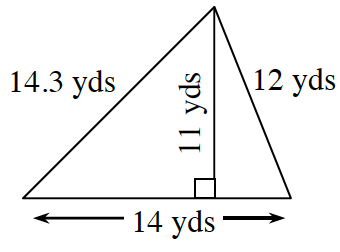2.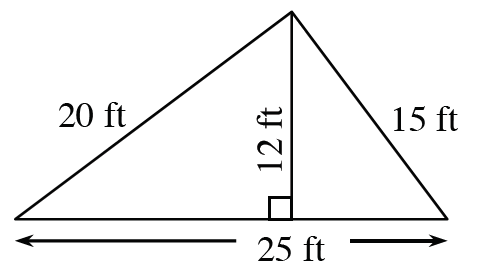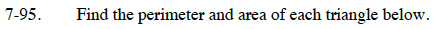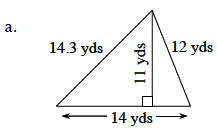The perimeter is the sum of all the sides of the shape.

14.3 + 12 + 14 = perimeter

Remember to include units.

The area of a triangle can be found using the formula:

$\text{Area = \frac{1}{2}(base)(height)}$

Remember to include units.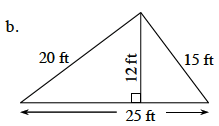See part (a).